# Independent Set Puzzles

In this post, I want to return to the idea of NP-Complete problems. There is a more technical, more formal definition that I can refer you to, but I like to refer to the images from Garey and Johnson’s “Computers and Intractability: A Guide to the Theory of NP Completeness”. The images helps to understand the difficulty of NP-Complete problems by presenting two images. The first image shows a single individual speaking to someone saying that he has been unable to solve the problem. The second image shows that same individual speaking to the same person behind the desk, but saying that not only was he unable to solve the problem but neither was a long line of people. The theory of NP Complete problems revolves around the concept that if an efficient algorithm exists for an NP-Complete problem, then an efficient algorithm exists for all problems in the class NP.

Today, I would like to present a puzzle I created to play with the Independent Set problem. This is the problem where we are given a graph G = (V, E) and are asked to find a maximum set of vertices S such that there is no edge in the graph G between any two vertices in S. The decision version (the problem asking whether there is an independent set of size k) of this problem is NP-Complete, so the known algorithms for problem either have a slow running time, or do not solve it exactly.

This problem is very related to another puzzle I posted last year called the clique problem. In fact, Karp originally proved that Clique was NP-Complete by showing that if Clique could be solved efficiently, then Independent Set could be solved efficiently. He did this by constructing a second graph [G bar], called the compliment of G (containing the same vertices in G, along with the edges that are not present in G. Any edge present in G will not be present in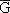). Then he showed that the nodes representing a maximum clique in G would represent a maximum independent set in. He had already shown that Independent Set was NP-Complete, which meant that both Independent Set and Clique were among the most difficult problems within the class known as NP.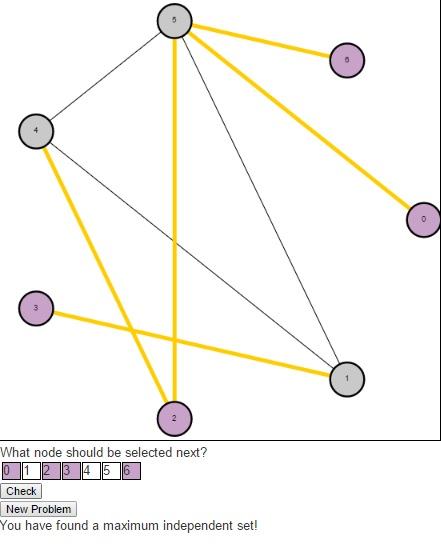The puzzle begins with an undirected graph and asks users to find a maximum independent set. Users should click on the numbers in the table below the graph indicating the nodes they wish to select in their independent set (purple indicates that the node is selected, gray indicates that it is not). Once a user have a potential solution, they can press the “Check” button to see if their solution is optimal. If a user is having trouble and simply wishes to see the maximum independent set, they can press the “Solve” button. And to generate a new problem, users can press the “New Problem” button.As a result of this relationship between the Clique problem and the Independent Set problem, the Bron-Kerbosch algorithm that was used to find maximum cliques previously can also be used here.

# Lets Learn About XOR Encryption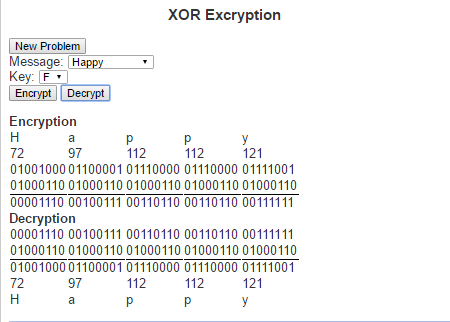One of the more common things about this generation is the constant desire to write up (type) their thoughts. So many of the conversations from my high school days were long lasting, but quickly forgotten. Today’s generation is much more likely to blog, tweet, write status updates or simply open up a notepad file and write up their thoughts after such a conversation.

When we feel that our thoughts are not ready for public eyes (maybe you want to run your idea by the Patent and Trademark Office before speaking about it) we may seek some form of security to ensure that they stay private. An old fashioned way of doing this was to write in a diary and enclosed it within a lock and key. The mathematical field of encryption also tries to grant privacy by encoding messages so that only people with the necessary information can read them.

The type of encryption I want to speak about today is called XOR encryption. It is based on the logical operation called “exclusive or” (hence the name XOR). The exclusive or operation is true between two logical statements if exactly one of the two statements is true, but not both statement. This can be represented with the following truth table

 Input 1 Input2 XOR Result T T F T F T F T T F F F

XOR Encryption is particularly useful in this day and age because we every character we type is understood by the computer as a sequence of zeros and ones. The current standard encoding that is used is Unicode (also known as UTF-8). Under this encoding the letter ‘a’ is represented as the binary string ‘01100001’. Similarly every letter, number and special character can be represented as its own binary string. These binary strings are just an assignment of numbers to these characters so that we can to help represent them in the computer. The numbers can the be thought of in base 10, which is how we generally think about numbers, or in base 2 which is how computers generally work with numbers (or a number of other ways). The way we would use these binary strings in encoding is first by translating a text from human-readable text to machine readable text via its binary string. For example, the word “Invincible”, we would get the following binary strings:

 Letter Unicode in base 10 Unicode in base 2 I 73 01001001 n 110 01101110 v 118 01110110 i 105 01101001 n 110 01101110 c 99 01100011 i 105 01101001 b 98 01100010 l 108 01101100 e 101 01100101

To encrypt the message we need a key to encode the message and will simply perform an XOR operation on the key and every character in the string. Similarly, do decrypt the message we perform XOR operation on the key and every character in the encoded message. This means that the key (much like a normal key to a diary) must be kept private and only those whom the message is to be shared between have access to it.

Here is a link to the script where you can check out XOR Encrpytio. Try it out and let me know what you think.

# Discrete-time Markov Chains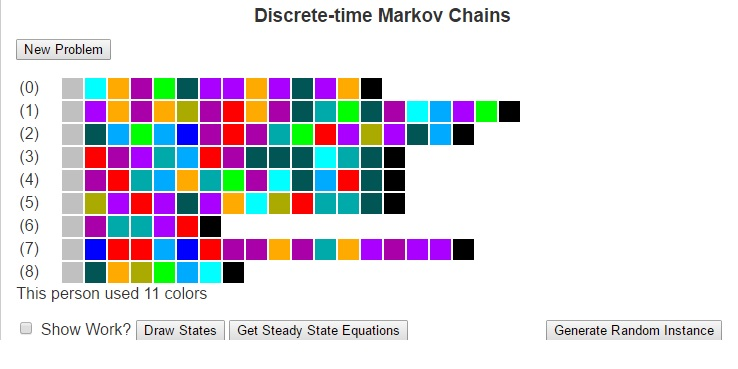Much of how we interact with life could be described as transitions between states. These states could be weather conditions (whether we are in a state of “sunny” or “rainy”), the places we may visit (maybe “school”, “the mall”, “the park” and “home”), our moods (“happy”, “angry”, “sad”). There are a number of other ways to model states and even the possibility of infinitely many states.

Markov Chains are based on the principle that the future is only dependent on the immediate past. so for example, if I wished to predict tomorrow’s weather using a Markov Chain, I would need to only look at the weather for today, and can ignore all previous data. I would then compare the state of weather for today with historically how weather has changed in between states to determine the most likely next state (i.e what the weather will be like tomorrow). This greatly simplifies the construction of models.

To use Markov Chains to predict the future, we first need to compute a transition matrix which shows the probability (or frequency) that we will travel from one state to another based on how often we have done so historically. This transition matrix can be calculated by looking at each element of the history as an instance of a discrete state, counting the number of times each transition occurs and dividing each result by the number of times the origin state occurs. I’ll next give an example and then I’ll focus on explaining the Finite Discrete State Markov Chain tool I built using javascript.

Next, I want to consider an example of using Markov Chains to predict the weather for tomorrow. Suppose that we have observed the weather for the last two weeks. We could then use that data to build a model to predict tomorrow’s weather. To do this, lets first consider some states of weather. Suppose that a day can be classified in one of four different ways: {Sunny, Cloudy, Windy, Rainy}. Further, suppose that over the last two weeks we have seen the following pattern.

 Day 1 Sunny Day 2 Sunny Day 3 Cloudy Day 4 Rain Day 5 Sunny Day 6 Windy Day 7 Rain Day 8 Windy Day 9 Rain Day 10 Cloudy Day 11 Windy Day 12 Windy Day 13 Windy Day 14 Cloudy

We can look at this data and calculate the probability that we will transition from each state to each other state, which we see below:

 Rain Cloudy Windy Sunny Rain 0 1/3 1/3 1/3 Cloudy 1/2 0 1/2 0 Windy 2/5 1/5 2/5 0 Sunny 0 1/3 1/3 1/3

Given that the weather for today is cloudy, we can look at the transition matrix and see that historically the days that followed a cloudy day have been Rainy and Windy days each with probability of 1/5. We can see this more mathematically by multiplying the current state vector (cloudy) [0, 1, 0, 0] by the above matrix, where we obtain the result [1/2, 0, 1/2, 0].

In similar fashion, we could use this transition matrix (lets call it T) to predict the weather a number of days in the future by looking at Tn. For example, if we wanted to predict the weather two days in the future, we could begin with the state vector [1/2, 0, 1/2, 0] and multiply it by the matrix T to obtain [1/5, 4/15, 11/30, 1/6].

We can also obtain this by looknig at the original state vector [0, 1, 0, 0] and multiplying it by T2.

T2 =

1

 3/10 8/45 37/90 1/9 1/5 4/15 11/30 1/6 13/50 16/75 59/150 2/15 3/10 8/45 37/90 1/9

When we multiply the original state vector by T2 we arrive at this same answer [1/5, 4/15, 11/30, 1/6]. This matrix T2 has an important property in that every state can reach every other state.

In general, if we have a transition matrix where for every cell in row i and column j, there is some power of the transition matrix such that the cell (i, j) in that matrx is nonzero, then we say that every state is reachable from every other state and we call the Markov Chain regular.

Regular Markov Chains are important because they converge to what’s called a steady state. These are state vectrs x = [x0, …, xn] such that xTn = x for very large values of n. The steady state tells us how the Markov Chain will perform over long periods of time. We can use algebra and systems of linear equations to solve for this steady state vector.

For the Javascript program I’ve written, I have generated a set of painting samples for a fictional artist. The states are the different colors and the transitions are the colors that the artist will use after other colors. as well as the starting and ending colors. Given this input, we can form a Markov Chain to understand the artist’s behavior. This Markov Chain can then be used to solve for the steady state vector or to generate random paintings according to the artist’s profile. Be sure to check it out and let me know what you think.

# Topological Sort

One of the things I generally say about myself is that I love learning. I can spend hours upon hours reading papers and algorithms to better understand a topic. Some of these topics are stand alone segments that I can understand in one sitting. Sometimes, however, there is a need to read up on some preliminary work in order to fully understand a concept.

Lets say that I was interested in organizing this information into a new course. The order I present these topics is very important. Knowing which topics depend on one another allows me to use the topological sorting algorithm to determine an ordering for the topics that respects the preliminary work.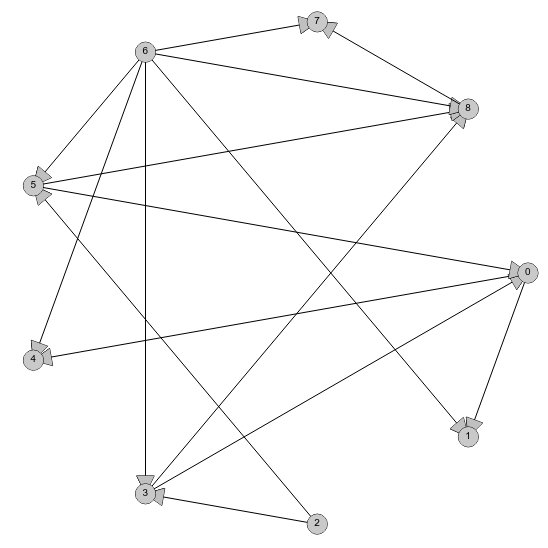The input for the topoligical sorting algorithm is a Directed Acyclic Graph (DAG). This is a set of relationships between pairs of topics, where if topic 1 must be understood before topic 2, we would add the relationship (topic 1, topic 2) to the graph. DAGs can be visualized by a set of nodes (points) representing the topics. Relationships like the one above (topic 1, topic 2) can then represented by a directed arc originating at topic 1 and flowing in the direction of topic 2. We say that the graph is “Acyclic” because there cannot be a cycle in the topic preliminaries. This amounts to us saying that a topic cannot be a prerequisite for itself. An example of a DAG is shown in the image above.

With the topics represented as a DAG, the topologial ordering algorithm works by searching the set of nodes for the one with no arcs coming into it. This node (or these nodes is multiple are present) represents the topic that can be covered next without losing understanding of the material. Such a node is guaranteed to exist by the acyclic property of the DAG. Once the node is selected, we can remove this node as well as all arcs that originate at this node from the DAG. The algorithm then repeats the procedure of searching for a nod with no arcs coming into it. This process repeats until there are no remaining nodes from which to choose.

Now lets see how the topological sort algorithm works on the graph above. We will first need to count the in-degree (the number of arcs coming into) each node.

Node | Indegree
—————-
0 | 2
1 | 2
2 | 0
3 | 2
4 | 2
5 | 2
6 | 0
7 | 2
8 | 3

Node to be removed (i.e. node with the minimum indegree): Node 2.
Arcs connected to node 2: (2, 5), (2, 3)
Resulting Indegree Count:
Node | Indegree
—————-
0 | 2
1 | 2
3 | 1
4 | 2
5 | 1
6 | 0
7 | 2
8 | 3

Node to be removed: Node 6:
Arcs connected to node 6: (6, 1), (6, 3), (6, 4), (6, 5), (6, 7), (6, 8)
Resulting Indegree Count:
Node | Indegree
—————-
0 | 2
1 | 1
3 | 0
4 | 1
5 | 0
7 | 1
8 | 2

Node to be removed: Node 3
Arcs connected to node 3: (3, 0), (3, 8)
Resulting Indegree Count:
Node | Indegree
—————-
0 | 1
1 | 1
4 | 1
5 | 0
7 | 1
8 | 1

Node to be removed: Node 5
Arcs connected to node 5: (5, 0), (5, 8)
Resulting Indegree Count:
Node | Indegree
—————-
0 | 0
1 | 1
4 | 1
7 | 1
8 | 0

Node to be removed: Node 0:
Arcs connected to node 0: (0, 1), (0, 4)
Resulting Indegree Count:
Node | Indegree
—————-
1 | 0
4 | 0
7 | 1
8 | 0

Node to be removed: Node 1
Arcs connected to node 1: none
Resulting Indegree Count:
Node | Indegree
—————-
4 | 0
7 | 1
8 | 0

Node to be removed: Node 4
Arcs connected to node 4: none
Resulting Indegree Count:
Node | Indegree
—————-
7 | 1
8 | 0

Node to be removed: Node 8
Arcs connected to node 8: (8, 7)
Resulting Indegree Count:
Node | Indegree
—————-
7 | 0

Node to be removed: Node 7
Arcs connected to node 7: none
Resulting Indegree Count:
Node | Indegree
—————-

Since there are no nodes remaining, we have arrived at a topological ordering. Going through this iteration, we can see that we arrived at the ordering (2, 6, 3, 5, 0, 1, 4, 8, 7). There were several occasions where there were multiple nodes with indegree of 0 and we could have selected an alternative node. This would have given us a different topological ordering of the nodes, but it would still be valid.

There are more learning opportunities and an interactive demonstration of the algorithm at Topological Sort Examples at LEARNINGlover.

# The RSA Algorithm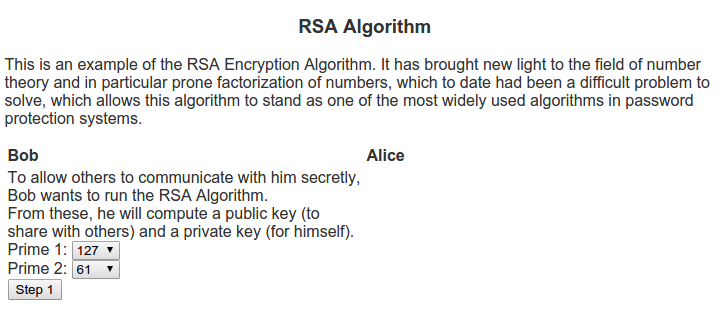I can remember back when I was in school, still deciding whether I wanted to study pure or applied mathematics. One of the common questions I would receive from those in applied mathematical realms would sound like “What’s the point of doing mathematics with no real world applications?”. Generally my response to these questions was about the intrinsic beauty of mathematics, no different from an artist painting not for some desire to be a millionaire, but because of an burning desire to paint. Whether their paintings would one day be on the walls of a Smithsonian museum or sit on their mother’s refrigerator is generally outside of the thought process of the artist. So too, would I argue about the thought process of a pure mathematician.

When I was an undergrad and learned about the RSA algorithm (named for Ron Rivest, Adi Shamir, and Leonard Adleman who discovered the algorithm) it helped me explain this concept a lot better. The algorithm is based on prime numbers and the problem of finding the divisors of a given number. Many mathematicians throughout the ages have written papers on the beauty of prime numbers (see Euclid, Eratosthenes, Fermat, Goldbach, etc). For a large period in time one of the beautiful things about prime numbers was that they were so interesting in themselves. There were questions about how to check if a number is prime, question of patterns in primes, famous conjectures like the Goldbach conjecture and the twin prime conjecture, quick ways of finding prime numbers or numbers that are almost always prime, etc. In short, this was an active area of research that much of the applied world was not using. This all changed in 1977 when Rivest, Shamir and Adleman published the RSA algorithm.

The algorithm is in the area called public key cryptography. These algorithms differ from many of the previous cryptography algorithms, namely symmetric key cryptography. Whereas symmetric key cryptography depends uses the same device (key) to encode as to decode, public key cryptography creates two keys – one for encoding that is generally shared with others, and another for decoding which is kept private. These two keys in generally relate to a mathematical problem that is very difficult to solve.

In my example script for the RSA Algorithm, I show two people who want to communicate, Alice and Bob. Bob wants people to be able to send him messages securely so he decides to use the RSA algorithm. To do this, he first needs to think of two prime numbers, p1 and p2.
From these, he computes the following:
n = p1 * p2

Next, he computes Euler’s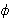function on this n which can be calculated as(n) = (p1 – 1) * (p2 – 1)

Then Bob looks for a number e that is relatively prime to. This is what he will use as the encryption key.

One he has e, he can calculate d, which is the multiplicative inverse of e in
(n).
This means that e * d = 1 (mod(n)).

The public key that will be used for encryption will be the pair (e, n). This is what he posts publicly via his web page for others to communicate with him securely. Bob also has a private key pair (d, n) that he will use to decrypt messages.

Alice sees Bob’s public key and would like to communicate with him. So she uses it to encode a message. The formula she uses to encrypt her message is c = me mod n, where c is the encrypted message. Once Alice encrypts her message, she sends it to Bob.

Bob receives this encoded message and uses the private key (d, n) to decode the message from Alice. The formula to decrypt is m = cd mod n.

For a more illustrative idea of how this algorithm works as well as examples, be sure to visit Script for the RSA Algorithm.